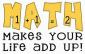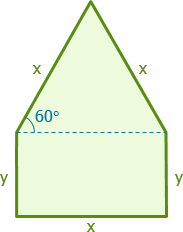# help: window consist of a rectangle with an equilateral triangle resting on its top

3 posts / 0 new
Juny Geraldizo ...help: window consist of a rectangle with an equilateral triangle resting on its top

pls help me to solve this problems as well as the solution:

1. a large window consist of a rectangle with an equilateral triangle resting on its top. if the perimeter P of the window is fixed at 33 ft, find the dimension of the triangle that will maximize the area of the window.

2. A student club on a college charges monthly membership dues of P10, less 5 centavos for each member over 60. How many members would give the club, the most revenue from the monthly dues?

Jhun VertSolution to #1
$3x + 2y = 33$

$y = \frac{33}{2} - \frac{3}{2}x$$A = xy + \frac{1}{2}x^2 \sin 60^\circ$

$A = x \left( \frac{33}{2} - \frac{3}{2}x \right) + \frac{1}{4}\sqrt{3}x^2$

$A = \frac{33}{2}x - \frac{3}{2}x^2 + \frac{1}{4}\sqrt{3}x^2$

$A = \frac{33}{2}x - \left( \frac{3}{2} - \frac{1}{4}\sqrt{3} \right) x^2$

$\dfrac{dA}{dx} = \frac{33}{2} - 2\left( \frac{3}{2} - \frac{1}{4}\sqrt{3} \right) x = 0$

$\frac{33}{2} = 2\left( \frac{3}{2} - \frac{1}{4}\sqrt{3} \right) x$

$x = \dfrac{\frac{33}{2}}{2\left( \frac{3}{2} - \frac{1}{4}\sqrt{3} \right)}$

$x = 6 + \sqrt{3} = 7.732 ~ \text{ft}$

Juny Geraldizo ...thank you sir for the help. kanang #2 sir pwede ko mangayu ug idea on how to solve?
thank you again sir....

• Mathematics inside the configured delimiters is rendered by MathJax. The default math delimiters are $$...$$ and $...$ for displayed mathematics, and $...$ and $...$ for in-line mathematics.